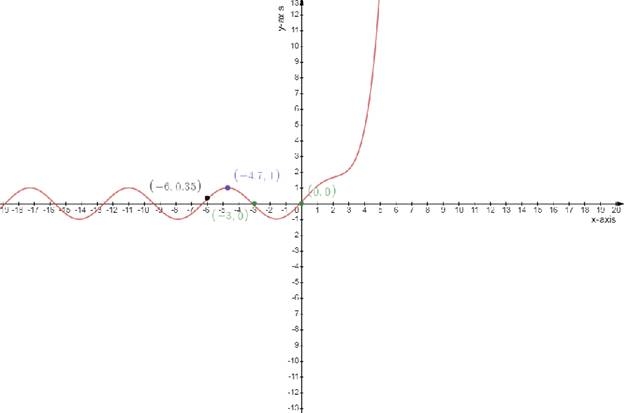# The antiderivative without using the chain rule### Single Variable Calculus: Concepts...

4th Edition
James Stewart
Publisher: Cengage Learning
ISBN: 9781337687805### Single Variable Calculus: Concepts...

4th Edition
James Stewart
Publisher: Cengage Learning
ISBN: 9781337687805

#### Solutions

Chapter 4, Problem 59RE

(a)

To determine

## To find:The antiderivative without using the chain rule

Expert Solution

F(x)=0.1

### Explanation of Solution

Given:

The function f(x)=0.1ex+sinx,4x4

F'(x)=f(x)

Concept used:

Antiderivative :- A function F(x) is antiderivative of on an interval I if F'(x)=f(x) for all x in interval I

The entire family of antiderivative of a function by adding a constant to a known antiderivative

So, if F(x) is the antiderivative of f(x) , then the family of the antiderivatives would be F(x)+c

Calculation:

The function f(x)=0.1ex+sinx,4x4.............(1)

if F'(x)=f(x)

Integrating the equation (1)

F(x)=0.1ex+sinxdxF(x)=0.1exdx+sinxdxF(x)=0.1exdx+sinxdxF(x)=0.1excosxF(0)=0.1e0cos(0)F(0)=0.1

(b)

To determine

Expert Solution

F(x)=0.1excosx

### Explanation of Solution

Given:

The function f(x)=0.1ex+sinx,4x4

F'(x)=f(x)

Concept used:

Antiderivative :- A function F(x) is antiderivative of on an interval I if F'(x)=f(x) for all x in interval I

The entire family of antiderivative of a function by adding a constant to a known antiderivative

So, if F(x) is the antiderivative of f(x) , then the family of the antiderivatives would be F(x)+c

Calculation:

The function f(x)=0.1ex+sinx,4x4.............(1)

if F'(x)=f(x)

Integrating the equation (1)

F(x)=0.1ex+sinxdxF(x)=0.1exdx+sinxdxF(x)=0.1exdx+sinxdxF(x)=0.1excosx

(c)

To determine

Expert Solution

### Explanation of Solution

Given:

The function is

f(x)=0.1ex+sinx,4x4

Concept used:

The slope of the tangent to a curve f(x) at any point a is given by the value of the first derivative of the slope at the point x=a .

The tangent to be horizontal so the slope should be equal to 0

That is dydx=0 .

Calculation:

The function is

f(x)=0.1ex+sinx,4x4................................(1)

Draw the table

f(x)=0.1ex+sinx,4x4

Test one point in each of the region formed by the graph

If the point satisfies the function then shade the entire region to denote that every point in the region satisfies the function

 x−axis 0 −3 −4.7 −6 y−axis 0 0 1 0.35

Draw the graph

f(x)=f(x)=0.1ex+sinx### Have a homework question?

Subscribe to bartleby learn! Ask subject matter experts 30 homework questions each month. Plus, you’ll have access to millions of step-by-step textbook answers!